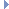﻿ MPointOnMesh Class Reference

# MPointOnMesh Class Reference

MPointOnMesh Class ReferenceRelated help topics:

`#include <MMeshIntersector.h>`

## Class Description

Mesh intersector result.

This class is used to return information about a point on a mesh: 3D position, normal, barycentric coordinates, etc. Note that this can be a point anywhere on the surface of the mesh, not just at vertices.Examples:

## Public Member Functions

MPointOnMesh ()
Constructor.

MFloatPointgetPoint ()
This method returns the point data.

MFloatVectorgetNormal ()
This method returns the normal data.

void getBarycentricCoords (float &u, float &v) const
This method returns the barycentric coordinates of the closest point on the mesh. More...

int faceIndex () const
This method returns the face index data.

int triangleIndex () const
This method returns the triangle index data.

## Friends

class MMeshIntersector

## Member Function Documentation

 void getBarycentricCoords ( float & u, float & v ) const

This method returns the barycentric coordinates of the closest point on the mesh.

If the triangle has vertices (a, b, c) then the point returned by getPoint() is at coordinates `u*a + v*b + (1 - u - v)*c`. The barycentric coordinates are particularly useful when interpolating attributes from one mesh to another.

Parameters
 [out] u The relative contribution of the first vertex of the triangle. [out] v The relative contribution of the second vertex of the triangle.

The documentation for this class was generated from the following files:
• MMeshIntersector.h
• MMeshIntersector.cpp